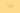Technically, duration is the weighted average length of time to the receipt of a bond’s benefits (coupons and redemption value) with the weightings being driven by the present value of the benefits involved. So the first year’s coupon of £5 only reduces the average life of the bond by a small amount. This approach is called Macaulay Duration.

The longer the duration of a bond, the more sensitive it is to changes in interest rates. Lower coupons and lower yields lengthen duration, which then begs the question: “What is the exact relationship to changes in interest rates and changes in the value of a bond?”

To answer that, we use what is called Modified Duration which calculates the percentage change in the price of a bond arising from a 1% change in yields. It is calculated by taking the Macaulay Duration and dividing it by (1 + [Gross Redemption Yield]).

Take a one year zero coupon bond and a three year zero coupon bond when interest rates are 2%, by way of example. The one year has a Modified Duration of 0.98% whereas the three year is at 2.94%. So, if interest rates rise by 1%, the three year bond will fall in price by 2.94%.